ChemTalk

# Bond Formation and Dissociation Energies## What is Bond Energy?

No molecule within chemistry would exist without bonds linking atoms. Because forming bonds releases energy, molecules exist because atoms prefer a stable compound with less energy. In order to break these stable bonds, energy must be added to the system. Thus, this value is known as bond energy.

Energy is always required when breaking a bonds as an endothermic reaction. On the other hand, energy is always released when forming bonds as an exothermic reaction.

Bond energies, or the strength of a bond, determine how much energy is released or required in these processes. Molecules can differ in their overall reaction enthalpies depending on its bonds.

## What Are Bond Formation and Dissociation Energies?

A molecule’s bond formation energy is the heat/energy released when forming the product. In a reaction, the bond formation energy is the energy released in the product-forming step. Consequently, this value is always negative as forming bonds release energy.

A molecule’s bond dissociation energy is the heat required to break bonds. In a reaction, the bond dissociation energy is the energy required to break apart the original reactant’s bonds. Consequently, this value is always positive as breaking bonds require energy.

Adding the dissociation and formation energies results in the overall enthalpy of reaction, or the net total of energy throughout the reaction’s progress. Thus, bond formation and dissociation energies determine whether an overall reaction’s enthalpy is endothermic or exothermic.

If breaking the reactant’s bonds require more energy than the energy released in the product’s formation, the reaction is endothermic. If a product’s formation releases more energy than breaking the reactant’s bonds, the reaction is exothermic.

## Bond Enthalpy Formula

Therefore, the bond energy formula helps calculate a reaction’s enthalpy based on bond dissociation and formation energies. The reaction below calculates the overall enthalpy as the bonds formed subtracted from bonds broken.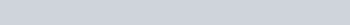In this equation: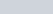overall reaction enthalpy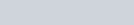sum of heat released by forming product’s bonds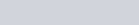sum of heat absorbed by breaking reactant’s bonds

This equation can solve for any of these three values and is a key formula in thermodyanamics, physical chemistry, and more.

## Bond Strength Qualities and Periodic Trends

Various qualities determine a bond’s dissociation and formation energies. Most commonly, a bond’s strength depends on:

• Bond Length
• Bond Order
• Bond Angle

Firstly, as shorter bonds share more valence electrons with other atoms, these bonds are often stronger and require more energy to break than longer bonds. As distance between the two atoms’ nuclei decreases, the bond strength increases.

Likewise as triple and double bonds are both shorter than a single bond, so multiple bonds have higher dissociation and formation energies. A higher bond order therefore results in more energy needed to break the bond.

Additionally, as a greater angle between atoms results in shorter bond lengths, molecules with larger angles require more energy to break its bonds. The opposite applies to molecules with a smaller angle between atoms.

As for periodic table trends related to bond formation/dissociation energies, atoms grow larger as you move down and left on the periodic table. As larger atomic radii result in longer bonds, these atoms often form bonds with smaller dissociation and formation energies. This periodic trend is vice versa for atoms with smaller atomic radii/bond lengths.

## Important Charts and Tables

The table below lists experimentally-determined average bond formation/dissociation energies for different bonds. All energy values are listed in terms of (kJ/mol)

Using the bond energy formula above, any value in the equation can be determined by adding the bond energies within a molecule.

## Bond Energy Equation Practice Problem

Finally, let’s try calculating the bond formation energy, dissociation energy, and overall reaction enthalpy with two practice problems!

For example, let’s consider the combustion of methane into carbon dioxide and water.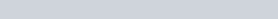Within this reaction, what is the overall reaction enthalpy? Similarly, is the reaction exothermic or endothermic?

In short, we can solve this problem by using the average bond energy table above and the bond enthalpy formula. On the reactants’ side, there are 4 C-H bonds and 2 O=O bonds. On the products’ side, there are 2 C=O bonds and 4 O-H bonds.